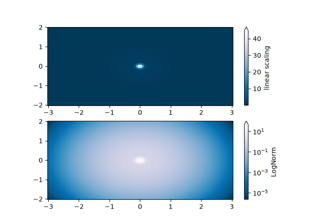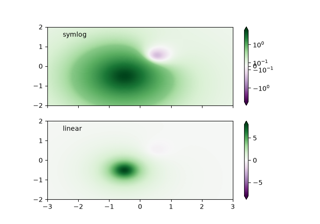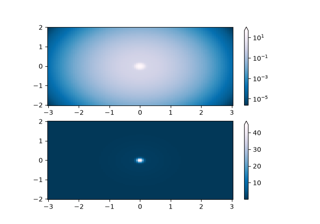# matplotlib.colors.SymLogNorm¶

class matplotlib.colors.SymLogNorm(linthresh, linscale=1.0, vmin=None, vmax=None, clip=False, *, base=10)[source]

The symmetrical logarithmic scale is logarithmic in both the positive and negative directions from the origin.

Since the values close to zero tend toward infinity, there is a need to have a range around zero that is linear. The parameter linthresh allows the user to specify the size of this range (-linthresh, linthresh).

Parameters: linthreshfloatThe range within which the plot is linear (to avoid having the plot go to infinity around zero). linscalefloat, default: 1This allows the linear range (-linthresh to linthresh) to be stretched relative to the logarithmic range. Its value is the number of decades to use for each half of the linear range. For example, when linscale == 1.0 (the default), the space used for the positive and negative halves of the linear range will be equal to one decade in the logarithmic range. basefloat, default: 10
__call__(value, clip=None)

Normalize value data in the [vmin, vmax] interval into the [0.0, 1.0] interval and return it.

Parameters: valueData to normalize. clipboolIf None, defaults to self.clip (which defaults to False).

Notes

If not already initialized, self.vmin and self.vmax are initialized using self.autoscale_None(value).

__init__(linthresh, linscale=1.0, vmin=None, vmax=None, clip=False, *, base=10)
Parameters: vmin, vmaxfloat or NoneIf vmin and/or vmax is not given, they are initialized from the minimum and maximum value, respectively, of the first input processed; i.e., __call__(A) calls autoscale_None(A). clipbool, default: FalseIf True values falling outside the range [vmin, vmax], are mapped to 0 or 1, whichever is closer, and masked values are set to 1. If False masked values remain masked. Clipping silently defeats the purpose of setting the over, under, and masked colors in a colormap, so it is likely to lead to surprises; therefore the default is clip=False.

Notes

Returns 0 if vmin == vmax.

__module__ = 'matplotlib.colors'
__slotnames__ = []
inverse(value)

## Examples using matplotlib.colors.SymLogNorm¶